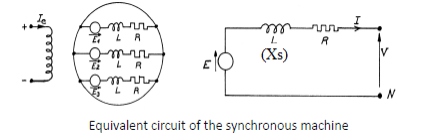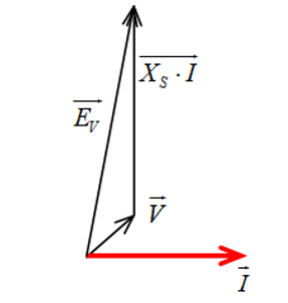# Power supply of an Airbus

EXERCISE : STUDY OF THE POWER SUPPLY OF AN AIRBUS A320

In flight, the electrical generation is ensured by two main alternators of 90 kVA which deliver a three-phase system of voltages 115V/200V, 400Hz. The frequency is kept constant thanks to a hydraulic regulation of the rotation speed of the alternators.We are interested in studying the unsaturated alternator. The aircraft’s electrical system is powered in 400 Hz. For the Airbus A320 the manufacturer gives:

 Rated voltage  VN/UN 115/200V Number of phases 3 Rated apparent power SN 90kVA Rated frequency fN 400Hz Rated speed nN 12.0×103rmp Power factor 0.75

The armature is star-coupled.  Two tests were performed at constant rated speed: nN
– Genrator at no-load test: no-load characteristic E0(Ie) where E0 is the value of induced electromotive force at no load in one winding and Ie the intensity of the inductive current. The
characteristic is a straight line such that for Ie=0 corresponds E0 = 0 and for Ie=92A corresponds E0=400 V.
Short-circuit test: in the useful range, the short-circuit characteristic is the line with equation  Isc = 3.07× Ie, where Isc is the rms value of the short-circuit current in a stator winding.
1- We are interested in nominal operation:
a) Calculate the pulsation of the output voltages of the alternator.       b) Determine the number of pole pairs of the machine.

c) Calculate the rms value of the nominal armature current IN.
2- We assume the alternator unsaturated.

a)  Drop its equivalent model by phase represented.   b) Calculate the synchronous impedance of the alternator Zs

c) Deduce the synchronous reactance Xs = Lsω

3- In the rest of the problem, the influence of stator resistance Rs is neglected.
a) Determine the intensity of the inductive current Ie0 for no-load operation at rated (nominal) voltage V=115 V.
b) We consider a balanced three-phase load. The alternator operates in nominal conditions; it delivers its rated current IN, behind (“en retard”) the voltage.
For cosφ = 0.75, represent the vector diagram of the voltages and deduce:
– the value of the e.m.f induced E0.    – the new value of the excitation current Ie for maintaining V = 115 V at cosφ= 0.75 when the alternator delivers its nominal current IN.

CORRECTION

1)

a) ω = 2πf = 25.1× 102 rd/s         b)  Ω = ω/p ⇒ p = 2        c) Sn = 3VnIn = √3UnIn ⇒ In  = 90×103/3×115 = 260A

2)  a)b) V = EZs.I  et Zs = EVCC/ICC  , The voltage EV evolves linearly with Ie and its equation is :  EV = 400×Ie/92   and  ICC = 400×Ie/92

Zs = EVCC/ICC  = (400×Ie/92 )/400×Ie/92  = (400/92)/3.07 = 1.4Ω             Xs = (Zs2 – Rs2) ≈1.4Ω

3)  a) For no-load operation at rated (nominal) voltage  V = 115V        V = Ev = 115V      EV = 400×Ie/92  so    Ie = (92/400)×115 = 26.5A

b) Vn = 115V       XsIN = 1.4× 260 = 364V     cosφ  = 0.75 →φv/I = 24.5° , on the diagram we read :  EV = 450V

We can verify by calculation : EV= [(vcosφ)2 + (Vsinφ XsI)2]½ = [(115cos41.5°)2 + (115sin41.5° + 364)2]½ = 448VEV = 450V      EV = 400×Ie/92  so    Ie = (92/400)×450  = 102A

Comments are closed.